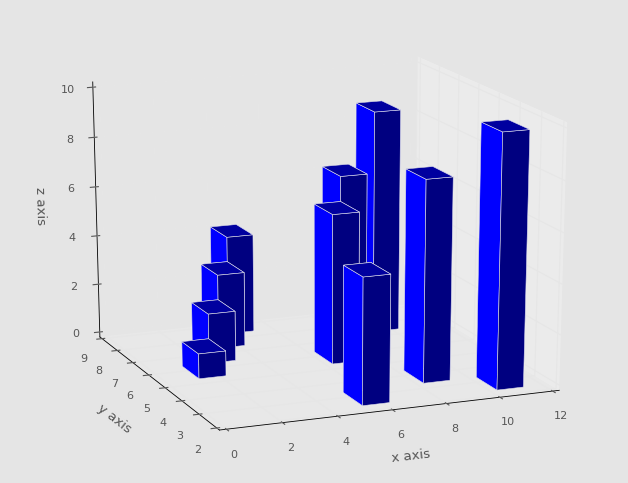## 3D Bar Chart with Matplotlib

In this Matplotlib tutorial, we cover the 3D bar chart. The 3D bar chart is quite unique, as it allows us to plot more than 3 dimensions. No, you cannot plot past the 3rd dimension, but you can plot more than 3 dimensions.

With bars, you have the starting point of the bar, the height of the bar, and the width of the bar. With a 3D bar, you also get another choice, which is depth of the bar. Most of the time, a bar chart starts with the bar flat on an axis, but you can add another dimension by releasing this constraint as well. We'll keep it rather simple, however:

```from mpl_toolkits.mplot3d import axes3d
import matplotlib.pyplot as plt
import numpy as np
from matplotlib import style
style.use('ggplot')

fig = plt.figure()

x3 = [1,2,3,4,5,6,7,8,9,10]
y3 = [5,6,7,8,2,5,6,3,7,2]
z3 = np.zeros(10)

dx = np.ones(10)
dy = np.ones(10)
dz = [1,2,3,4,5,6,7,8,9,10]

ax1.bar3d(x3, y3, z3, dx, dy, dz)

ax1.set_xlabel('x axis')
ax1.set_ylabel('y axis')
ax1.set_zlabel('z axis')

plt.show()```

Note here that we have to define x,y,z... and then 3 more dimensions for depth. This gives us:The next tutorial:• Introduction to Matplotlib and basic line

• Legends, Titles, and Labels with Matplotlib

• Bar Charts and Histograms with Matplotlib

• Scatter Plots with Matplotlib

• Stack Plots with Matplotlib

• Pie Charts with Matplotlib

• Data from the Internet for Matplotlib

• Converting date stamps for Matplotlib

• Basic customization with Matplotlib

• Unix Time with Matplotlib

• Colors and Fills with Matplotlib

• Spines and Horizontal Lines with Matplotlib

• Candlestick OHLC graphs with Matplotlib

• Styles with Matplotlib

• Live Graphs with Matplotlib

• Annotations and Text with Matplotlib

• Annotating Last Price Stock Chart with Matplotlib

• Subplots with Matplotlib

• Implementing Subplots to our Chart with Matplotlib

• More indicator data with Matplotlib

• Custom fills, pruning, and cleaning with Matplotlib

• Share X Axis, sharex, with Matplotlib

• Multi Y Axis with twinx Matplotlib

• Custom Legends with Matplotlib

• Basemap Geographic Plotting with Matplotlib

• Basemap Customization with Matplotlib

• Plotting Coordinates in Basemap with Matplotlib

• 3D graphs with Matplotlib

• 3D Scatter Plot with Matplotlib

• 3D Bar Chart with Matplotlib
• Conclusion with Matplotlib